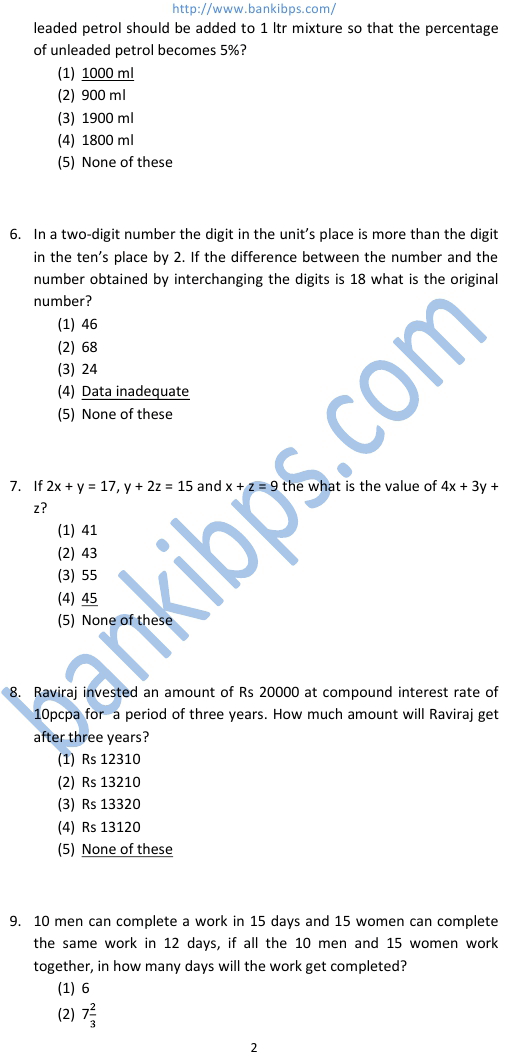# Federal Bank PO Question Papers

1. If 25% of a member is subtracted from a second number the second number reduces to its five-sixths. What is the ratio between the first number and the second number? (1) 2:3 (2) 3:2 (3) 1:3 (4) Data inadequate (5) None of these federal bank po question papers 2. Paramsehwaran invested an amount of Rs 12000 at the simple interest rate of 10% per annum and another amount at the simple interest rate of 20% per annum. The total interest earned at the end of one tear on the total amount invested became 14% per annum. Find the total amount invested? (1) Rs 22000 (2) Rs 25000 (3) Rs 20000 (4) Rs 24000 (5) None of these 3. Four of the five parts numbered (1), (2), (3), (4) and (5) in the following equation are exactly equal. Which part is not equal to the other four? The number of that part is the answer. (1) x(x + y) – 2x2y = (2) x(x – y)2 + 2x2y = (3) x(x2 + y2) = (4) x[(x + y)2 -2xy] = (5) x[(x + y)2 – 2xy2] 4. What will be the ratio between the area of a rectangle and the area of a triangle with one of the sides of rectangle as base and a vertex on the opposite side of rectangle. (1) 1:2 (2) 2:1 (3) 3:1 (4) Data inadequate (5) None of these
Practice Exercise - 351 [ Aptitude ]## federal bank po question papers

### federal bank po questions pdf

#### federal bank po interview questions

##### federal bank po exam question papers
###### federal bank po exam question
federal bank po question papers. federal bank po questions and answers. federal bank po questions. federal bank po questions pdf. federal bank po interview questions. federal bank po exam question papers. federal bank po exam question. federal bank po sample question papers. federal bank po solved question papers. federal bank po previous papers.